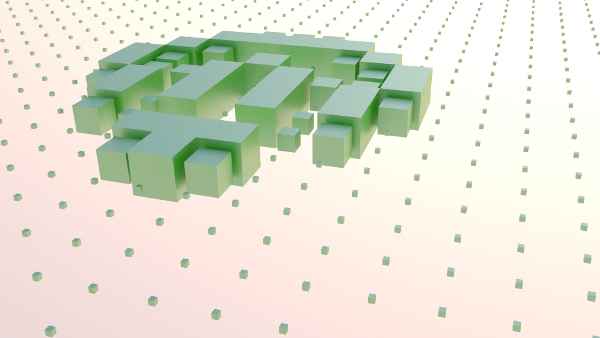## blender game of life

I implemented a game-of-life engine in python that generates blender animations

The generation of the blend file takes a while and they get quite big so I made the python script available at the end of the blog post instead of the blend file this timeimport the script in a new text-editor in blender and press run script.

``````import bpy

def run():
w = 40
f = 1
mat = bpy.data.materials['Material']
bpy.ops.anim.change_frame( frame = f )

cubes = [[ 0 for i in range(w)] for j in range(w)]
for x in range(w):
for y in range(w):
bpy.ops.mesh.primitive_cube_add( location=(x * 2, y * 2, 0 ))
cubes[x][y] = bpy.context.active_object
cubes[x][y].data.materials.append( mat );
cubes[x][y].scale=(.1,.1,.1)

cells = [[ 0 for i in range(w)] for j in range(w)]
nextGen = [[ 0 for i in range(w)] for j in range(w)]

cells = 1
cells = 1
cells = 1
cells = 1
cells = 1
cells = 1
cells = 1
cells = 1

for l in range(50):
f += 5
bpy.ops.anim.change_frame( frame = f )
for x in range(w):
row = ""
for y in range(w):
nb = 0
for i in range(-1,2):
for j in range( -1, 2):
xx = (x + i + w) % w
yy = (y + j + w) % w

if not( xx == x and yy == y):
nb += cells[xx][yy]

if ( cells[x][y] == 1 and (nb == 2 or nb == 3)):
nextGen[x][y] = 1
elif ( cells[x][y] == 0 and nb == 3 ):
nextGen[x][y] = 1
else:
nextGen[x][y] = 0

n = cubes[x][y]
bpy.context.scene.objects.active = n
n.select = True
if  cells[x][y] == 1 :
#row += 'X'
cubes[x][y].scale=(1,1,1)
else:
#row += '.'
cubes[x][y].scale=(.1,.1,.1)

for x in range( w ):
for y in range(w):
cells[x][y] = nextGen[x][y]

if __name__ == "__main__":
run();
``````

Leave a response

1. Hey!

Very nice tutorial.

The code apparently need a modification for running on the last version of Blender.